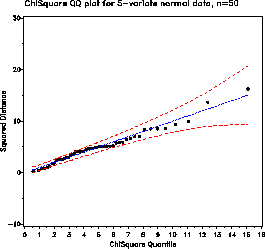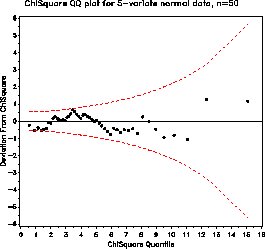cqplot Chi-square Q-Q plot cqplot

# SAS Macro Programs: cqplot

\$Version: 1.2 (09 Dec 2003)
Michael Friendly
York University

## The cqplot macro (get cqplot.sas)

The cqplot macro produces quantile-quantile comparison plots for multivariate normal data (based on squared Mahalanobis distances from the centroid) or for any other data which should follow a Chi-square distribution, together with estimated confidence bands.

For p variables and a large sample size, the squared Mahalanobis distances of the observations to the mean vector are distributed as chi-square with p degrees of freedom when the data has a multivariate normal distribution. However, the sample size must be quite large for the chi-square distribution to obtain unless p is very small. Also, this plot is sensitive to the presence of outliers. So, this plot should be cautiously used as a rough indicator of multivariate normality.

The macro produces either printer plots or high-resolution plots, or both. In addition, the macro can plot a detrended version, in which the vertical axis shows the difference between the data value and the expected Chi-square value.

### Method

Confidence intervals for the Chi-square QQ plot are based on standard error formulas given by J. Chambers et al. Graphical methods for data analysis, 1983.

### Usage

cqplot is a macro program. Values must be supplied for the VAR= variables, or supply a value for the DSQ= parameter.
```   %cqplot(data=inputdataset, var=inputvariables, ..., )
```
DATA=_LAST_
The name of the input dataset. If not specified, the most recently created dataset is used.
VAR=
Names of the variables whose squared distances are to be plotted
ID=
The name of an ID variable, used to label observations which fall outside the upper confidence band.
NVAR=
The number of variables in the VAR= list
DSQ=
If the input dataset already contains a chi-square variable to be plotted, specify the name of this variable as the DSQ= parameter.
PPLOT=NO
Produce printer plots?
GPLOT=YES
Produce Hi-res (SAS/GRAPH) plots?
OUT=cqplot
Name of the output data set
STDERR=YES
plot std errors around curves?
STDMULT=2
Multiplier of the standard error used for confidence bands.
DETREND=YES
plot detrended version?
LH=1.5
height for axis labels
ANNO=
The name of an optional input annotate data set which can be used to add graphical information (observation labels, etc.) to the QQ plot.
ANNOD=
The name of an optional input annotate data set which can be used to add graphical information (observation labels, etc.) to the detrended QQ plot.
NAME=CQPLOT
The name of graphic catalog entries
GOUT=
The name of graphic catalog in which hi-res plots are stored.

### Example

This example generates random 5-variate data from a multivariate normal distribution.
```%let dist=normal;
%let nobs=50;
%let nvar=5;

title "ChiSquare QQ plot for &nvar-variate &dist data, n=&nobs";
data cqtest;
drop i;
do i=1 to &nobs;
x1 = &dist(123425535);
x2 = &dist(123425535) + x1;
x3 = &dist(123425535) + x1 - x2;
x4 = &dist(123425535) - x1 + x2;
x5 = &dist(123425535) - x1 + x2 + x3;
output;
end;
```
The cqplot macro plots the squared distances of each observation from the centroid.
```%cqplot(data=cqtest, var=x1-x5, nvar=5);
```
The macro call produces these two plots:You can see what happens with non-normal data by changing the line
```%let dist=normal;
```
to
```%let dist=uniform;    /* Uniform distribution */
```
or
```%let dist=rancau;    /* Cauchy distribution */
```

### See also

nqplot Normal QQ plot
normplot
outlier Robust multivariate outlier detection